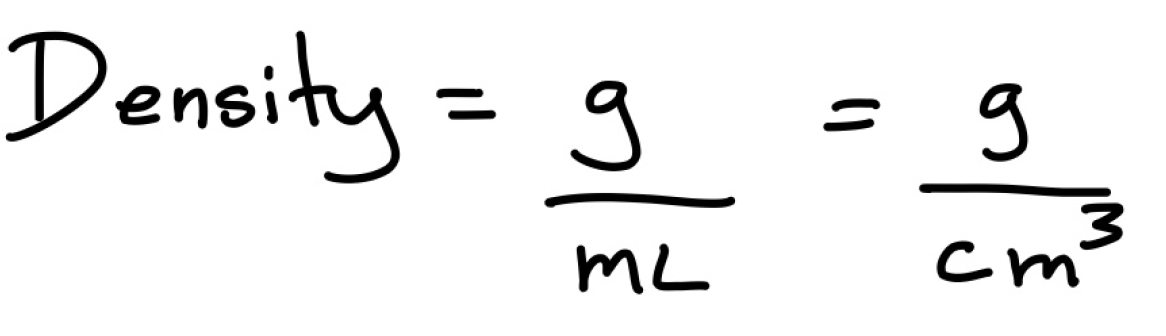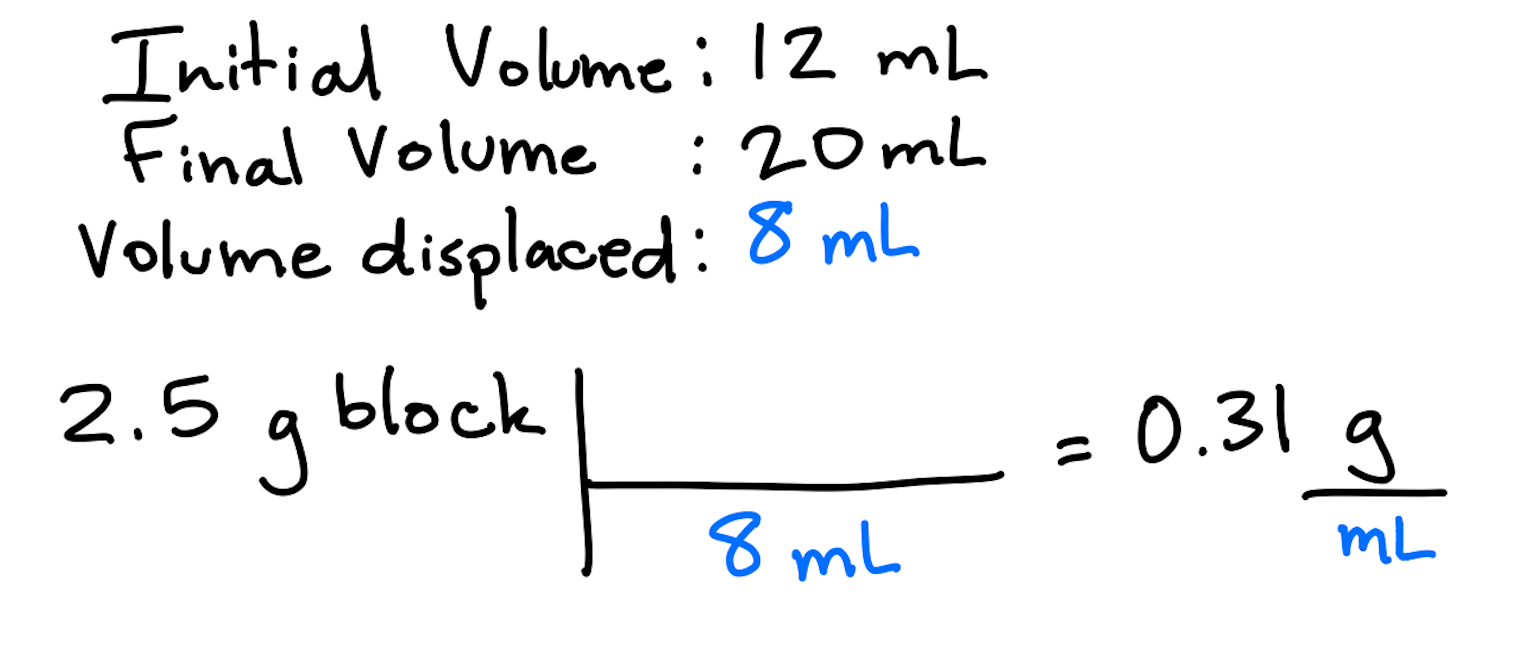Cyber Monday Sale: ALL MCAT Products & Admissions Services

### Density

Topic: Stoichiometry

The density of an object represents its “compactness”, or mass per given volume of a substance, and is most commonly represented as grams per milliliters, or g/mL.

The ratio of mass to volume determines how dense or “tightly packed” an object is; the higher the density value, the larger its mass is compared to the amount of space it occupies. A penny sinks in water due to its mass being proportionally larger than the space it occupies; a ship floats due to the large amount of space it takes up compared to its mass.

The unit for density, g/mL, can be written in many different ways because of its compatibility with other SI units:To calculate the density of an object in g/mL, the mass of the object can be taken in grams. In a situation where the object can be submerged in a liquid, the volume displacement can be calculated; this value can be found by subtracting the initial volume of the liquid from the final volume in mL:To calculate the density of an object in g/cm3, the grams are found by measuring the mass of the object and the volume of the object is found from its dimensions. For example, to find the volume of a rectangular block without using liquid we can do the following calculation:Notice how the unit of centimeters cubed (cm3) is a result of multiplying the units of the length, width, and height of the block together.

Practice Questions

MCAT Official Prep (AAMC)

Chemistry Online Flashcards Question 11

Sample Test C/P Section Question 26

Sample Test C/P Section Passage 7 Question 34

Key Points

• Density describes how compact an object is and can be defined as g/mL.

• For calculating the density, volume in mL can be found by calculating the volume displacement of a liquid after the object of interest is immersed in it.

• Volume in cmcan be found by multiplying dimensions in cm (length x width x height).

Key Terms

Compact: closely packed together.

Volume displacement: change in initial and final volume reading when an object is placed into a liquid (Vfinal-Vinitial) .

Billing Information